# Plotting and Visualization in Python¶

In 2016, there are more options for generating plots in Python than ever before:

• matplotlib
• Pandas
• Seaborn
• ggplot
• Bokeh
• Altair
• Plotly

These packages vary with respect to their APIs, output formats, and complexity. A package like matplotlib, while powerful, is a relatively low-level plotting package, that makes very few assumptions about what constitutes good layout (by design), but has a lot of flexiblility to allow the user to completely customize the look of the output.

On the other hand, Seaborn and Pandas include methods for DataFrame and Series objects that are relatively high-level, and that make reasonable assumptions about how the plot should look. This allows users to generate publication-quality visualizations in a relatively automated way.

# Matplotlib¶

Matplotlib is an excellent 2D and 3D graphics library for generating scientific figures in Python. Some of the many advantages of this library includes:

• Easy to get started
• Support for $\LaTeX$ formatted labels and texts
• Great control of every element in a figure, including figure size and DPI.
• High-quality output in many formats, including PNG, PDF, SVG, EPS.
• GUI for interactively exploring figures and support for headless generation of figure files (useful for batch jobs).

One of the of the key features of matplotlib that I would like to emphasize, and that I think makes matplotlib highly suitable for generating figures for scientific publications is that all aspects of the figure can be controlled programmatically. This is important for reproducibility, convenient when one need to regenerate the figure with updated data or changes its appearance.

In :
%matplotlib inline
import pandas as pd
import numpy as np
import matplotlib.pyplot as plt


## MATLAB-like API¶

As its name suggests, matplotlib is designed to compatible with MATLAB's plotting functions, so it is easy to get started with if you are familiar with MATLAB.

### Example¶

Let's import some data and plot a simple figure with the MATLAB-like plotting API.

In :
rain = pd.read_table('../data/nashville_precip.txt', delimiter='\s+', na_values='NA', index_col=0)

Out:
Jan Feb Mar Apr May Jun Jul Aug Sep Oct Nov Dec
Year
1871 2.76 4.58 5.01 4.13 3.30 2.98 1.58 2.36 0.95 1.31 2.13 1.65
1872 2.32 2.11 3.14 5.91 3.09 5.17 6.10 1.65 4.50 1.58 2.25 2.38
1873 2.96 7.14 4.11 3.59 6.31 4.20 4.63 2.36 1.81 4.28 4.36 5.94
1874 5.22 9.23 5.36 11.84 1.49 2.87 2.65 3.52 3.12 2.63 6.12 4.19
1875 6.15 3.06 8.14 4.22 1.73 5.63 8.12 1.60 3.79 1.25 5.46 4.30
In :
x = rain.index.values
y = rain['Jan'].values

In :
plt.figure()
plt.plot(x, y, 'r')
plt.xlabel('Year')
plt.ylabel('Rainfall')
plt.title('January rainfall in Nashville')

Out:
<matplotlib.text.Text at 0x112624fd0>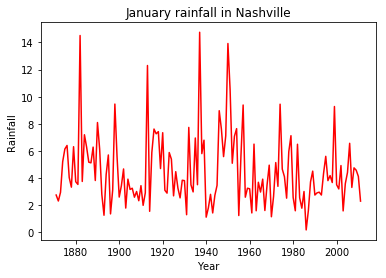It is straightforward to customize plotting symbols and create subplots.

In :
plt.figure(figsize=(14,6))
plt.subplot(1,2,1)
plt.plot(x, y, 'r--')
plt.subplot(1,2,2)
plt.plot(x, rain['Feb'], 'g*-')

Out:
[<matplotlib.lines.Line2D at 0x112c40860>]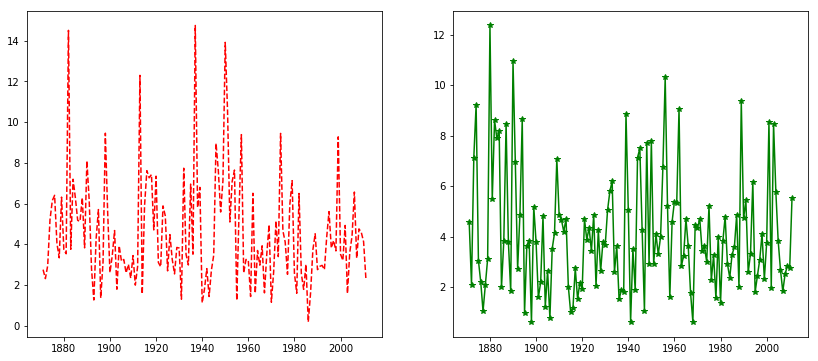While the MATLAB-like API is easy and convenient, it is worth learning matplotlib's object-oriented plotting API. It is remarkably powerful and for advanced figures, with subplots, insets and other components it is very nice to work with.

## Object-oriented API¶

The main idea with object-oriented programming is to have objects with associated methods and functions that operate on them, and no object or program states should be global.

To use the object-oriented API we start out very much like in the previous example, but instead of creating a new global figure instance we store a reference to the newly created figure instance in the fig variable, and from it we create a new axis instance axes using the add_axes method in the Figure class instance fig.

In :
fig = plt.figure()

# left, bottom, width, height (range 0 to 1)
# as fractions of figure size
axes = fig.add_axes([0.1, 0.1, 0.8, 0.8])

axes.plot(x, y, 'r')

axes.set_xlabel('Year')
axes.set_ylabel('Rainfall')
axes.set_title('January rainfall in Nashville');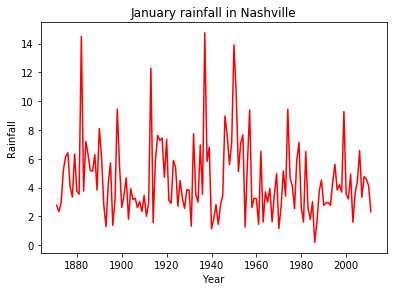Although a little bit more code is involved, the advantage is that we now have full control of where the plot axes are place, and we can easily add more than one axis to the figure.

In :
fig = plt.figure()

axes1 = fig.add_axes([0.1, 0.1, 0.9, 0.9]) # main axes
axes2 = fig.add_axes([0.65, 0.65, 0.3, 0.3]) # inset axes

# main figure
axes1.plot(x, y, 'r')
axes1.set_xlabel('Year')
axes1.set_ylabel('Rainfall')
axes1.set_title('January rainfall in Nashville');

# insert
axes2.plot(x, np.log(y), 'g')
axes2.set_title('Log rainfall');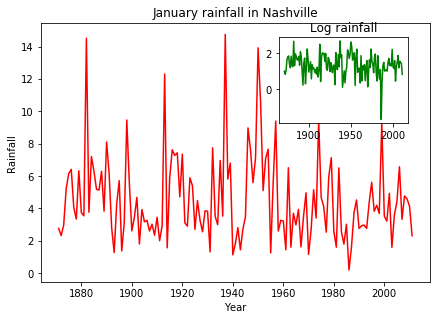If we don't care to be explicit about where our plot axes are placed in the figure canvas, then we can use one of the many axis layout managers in matplotlib, such as subplots.

In :
fig, axes = plt.subplots(nrows=4, ncols=1)

months = rain.columns

for i,ax in enumerate(axes):
ax.plot(x, rain[months[i]], 'r')
ax.set_xlabel('Year')
ax.set_ylabel('Rainfall')
ax.set_title(months[i])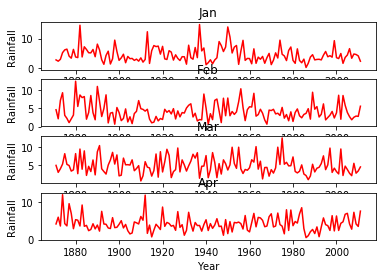That was easy, but it's not so pretty with overlapping figure axes and labels, right?

We can deal with that by using the fig.tight_layout method, which automatically adjusts the positions of the axes on the figure canvas so that there is no overlapping content:

In :
fig, axes = plt.subplots(nrows=4, ncols=1, figsize=(10,10))

for i,ax in enumerate(axes):
ax.plot(x, rain[months[i]], 'r')
ax.set_xlabel('Year')
ax.set_ylabel('Rainfall')
ax.set_title(months[i])

fig.tight_layout()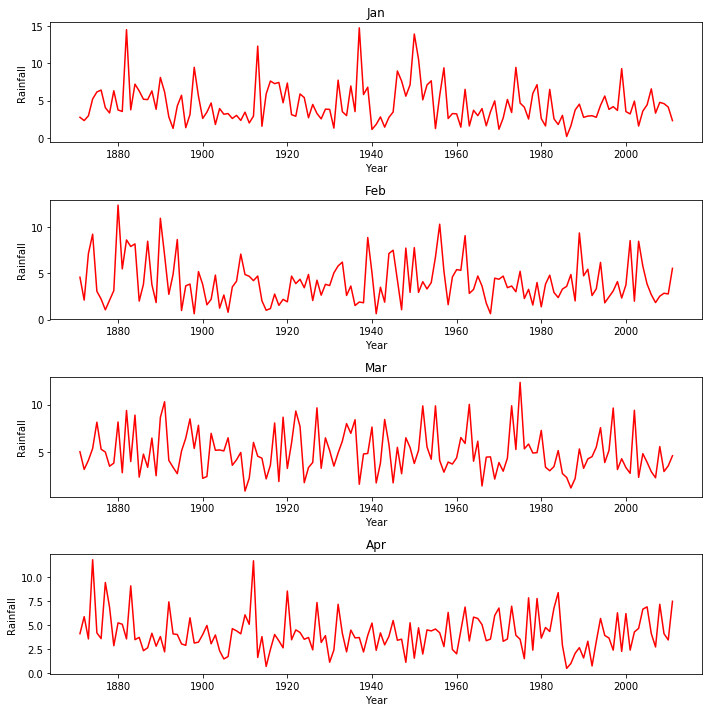## Manipulating figure attributes¶

Matplotlib allows the aspect ratio, DPI and figure size to be specified when the Figure object is created, using the figsize and dpi keyword arguments. figsize is a tuple with width and height of the figure in inches, and dpi is the dot-per-inch (pixel per inch). To create a figure with size 800 by 400 pixels we can do:

In :
fig = plt.figure(figsize=(8,4), dpi=100)

<matplotlib.figure.Figure at 0x112eed908>

The same arguments can also be passed to layout managers, such as the subplots function.

In :
fig, axes = plt.subplots(figsize=(12,3))

axes.plot(x, y, 'r')
axes.set_xlabel('Year')
axes.set_ylabel('Rainfall')

Out:
<matplotlib.text.Text at 0x1138a5b00>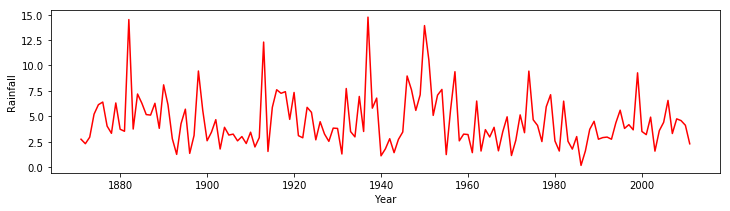Legends can also be added to identify labelled data.

In :
fig, ax = plt.subplots(figsize=(12,3))

ax.plot(x, rain['Jan'], label="Jan")
ax.plot(x, rain['Aug'], label="Aug")
ax.set_xlabel('Year')
ax.set_ylabel('Rainfall')
ax.legend(loc=1); # upper left corner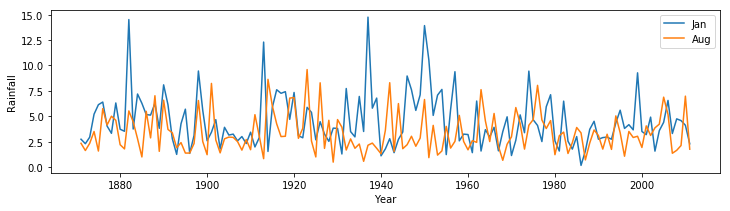Visualizations can be fine tuned in maplotlib, using the attibutes of the figure and axes.

In :
fig = plt.figure(figsize=(3.54, 3.2))

muy = [0.1,0.15,0.2,0.25,0.3,0.35,0.4,0.52,0.54]
sx = [5.668743677,9.254533132,14.23590137,11.87910853,14.6118157,16.8120231,18.58892361,100.1652558,443.4712272]
er = [0.986042277,1.328704279,0.913025089,0.997960015,1.921483929,4.435,2.817,0,0]

gensub = np.arange(0, 25, 0.1)
mon = [0.2386*np.log(i)-0.3751 for i in gensub]

ax.set_ylabel("$\mu$ (h$^{-1}$)")
ax.set_xlabel("S ($\mu$M)")

ax.set_xticks(np.arange(5, 23, 2))
ax.set_xlim(4.5, 21.5)

ax.set_yticks(np.arange(0.1, 0.5, 0.1))
ax.set_ylim(0, 0.5)

ax.errorbar(sx, muy, xerr=er, barsabove=True, ls="none", marker="o", mfc="w", color="k")
ax.plot(gensub, mon, ls="dotted", marker="None", mfc="k", color="k")

/Users/fonnescj/anaconda3/envs/dev/lib/python3.6/site-packages/ipykernel_launcher.py:9: RuntimeWarning: divide by zero encountered in log
if __name__ == '__main__':

Out:
[<matplotlib.lines.Line2D at 0x113e33f60>]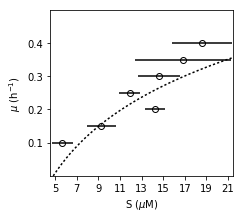# Plotting with Pandas¶

matplotlib is a relatively low-level plotting package, relative to others. It makes very few assumptions about what constitutes good layout (by design), but has a lot of flexiblility to allow the user to completely customize the look of the output.

On the other hand, Pandas includes methods for DataFrame and Series objects that are relatively high-level, and that make reasonable assumptions about how the plot should look.

In :
normals = pd.Series(np.random.normal(size=10))
normals.plot()

Out:
<matplotlib.axes._subplots.AxesSubplot at 0x113ce6e10>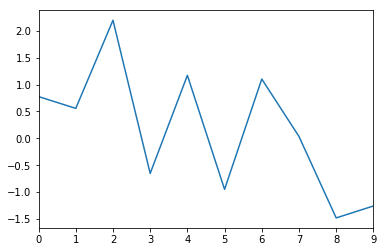Notice that by default a line plot is drawn, and light background is included. These decisions were made on your behalf by pandas.

All of this can be changed, however:

In :
normals.cumsum().plot(grid=True)

Out:
<matplotlib.axes._subplots.AxesSubplot at 0x1135df0b8>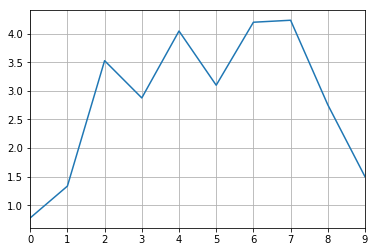Similarly, for a DataFrame:

In :
variables = pd.DataFrame({'normal': np.random.normal(size=100),
'gamma': np.random.gamma(1, size=100),
'poisson': np.random.poisson(size=100)})
variables.cumsum(0).plot()

Out:
<matplotlib.axes._subplots.AxesSubplot at 0x113d27b70>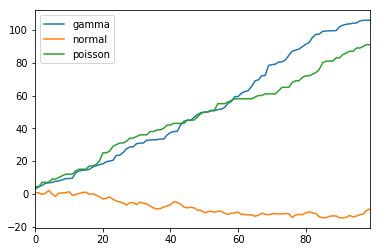As an illustration of the high-level nature of Pandas plots, we can split multiple series into subplots with a single argument for plot:

In :
variables.cumsum(0).plot(subplots=True, grid=True)

Out:
array([<matplotlib.axes._subplots.AxesSubplot object at 0x113169080>,
<matplotlib.axes._subplots.AxesSubplot object at 0x112eedb38>,
<matplotlib.axes._subplots.AxesSubplot object at 0x112c72588>], dtype=object)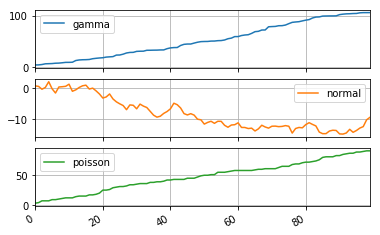Or, we may want to have some series displayed on the secondary y-axis, which can allow for greater detail and less empty space:

In :
variables.cumsum(0).plot(secondary_y='normal', grid=True)

Out:
<matplotlib.axes._subplots.AxesSubplot at 0x113852c50>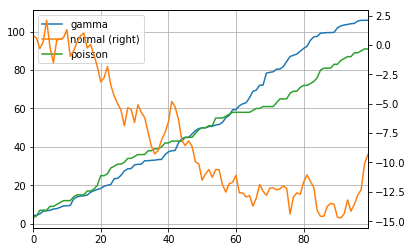If we would like a little more control, we can use matplotlib's subplots function directly, and manually assign plots to its axes:

In :
fig, axes = plt.subplots(nrows=1, ncols=3, figsize=(12, 4))
for i,var in enumerate(['normal','gamma','poisson']):
variables[var].cumsum(0).plot(ax=axes[i], title=var)
axes.set_ylabel('cumulative sum')

Out:
<matplotlib.text.Text at 0x112edb358>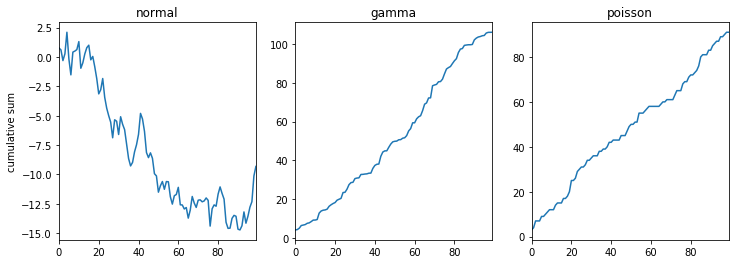## Bar plots¶

Bar plots are useful for displaying and comparing measurable quantities, such as counts or volumes. In Pandas, we just use the plot method with a kind='bar' argument.

For this series of examples, let's load up the Titanic dataset:

In :
titanic = pd.read_excel("../data/titanic.xls", "titanic")

Out:
pclass survived name sex age sibsp parch ticket fare cabin embarked boat body home.dest
0 1 1 Allen, Miss. Elisabeth Walton female 29.0000 0 0 24160 211.3375 B5 S 2 NaN St Louis, MO
1 1 1 Allison, Master. Hudson Trevor male 0.9167 1 2 113781 151.5500 C22 C26 S 11 NaN Montreal, PQ / Chesterville, ON
2 1 0 Allison, Miss. Helen Loraine female 2.0000 1 2 113781 151.5500 C22 C26 S NaN NaN Montreal, PQ / Chesterville, ON
3 1 0 Allison, Mr. Hudson Joshua Creighton male 30.0000 1 2 113781 151.5500 C22 C26 S NaN 135.0 Montreal, PQ / Chesterville, ON
4 1 0 Allison, Mrs. Hudson J C (Bessie Waldo Daniels) female 25.0000 1 2 113781 151.5500 C22 C26 S NaN NaN Montreal, PQ / Chesterville, ON
In :
titanic.groupby('pclass').survived.sum().plot.bar()

Out:
<matplotlib.axes._subplots.AxesSubplot at 0x1152756a0>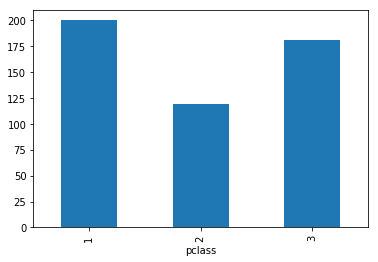In :
titanic.groupby(['sex','pclass']).survived.sum().plot.barh()

Out:
<matplotlib.axes._subplots.AxesSubplot at 0x115234e80>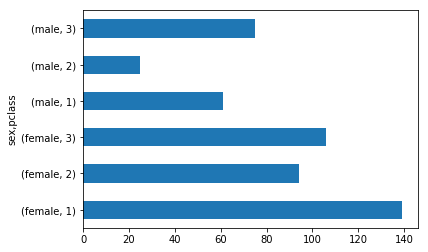In :
death_counts = pd.crosstab([titanic.pclass, titanic.sex], titanic.survived.astype(bool))
death_counts.plot.bar(stacked=True, color=['black','gold'], grid=True)

Out:
<matplotlib.axes._subplots.AxesSubplot at 0x1132d08d0>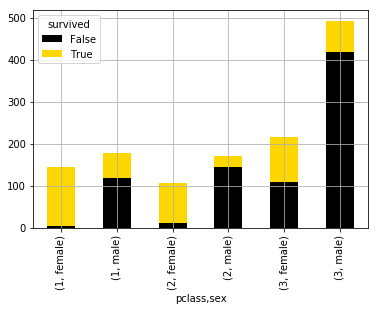Another way of comparing the groups is to look at the survival rate, by adjusting for the number of people in each group.

In :
death_counts.div(death_counts.sum(1).astype(float), axis=0).plot.barh(stacked=True, color=['black','gold'])

Out:
<matplotlib.axes._subplots.AxesSubplot at 0x1155109b0>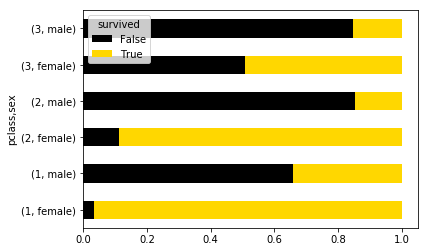## Histograms¶

Frequenfly it is useful to look at the distribution of data before you analyze it. Histograms are a sort of bar graph that displays relative frequencies of data values; hence, the y-axis is always some measure of frequency. This can either be raw counts of values or scaled proportions.

For example, we might want to see how the fares were distributed aboard the titanic:

In :
titanic.fare.hist(grid=False)

Out:
<matplotlib.axes._subplots.AxesSubplot at 0x11566bc50>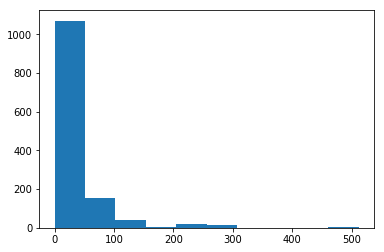The hist method puts the continuous fare values into bins, trying to make a sensible décision about how many bins to use (or equivalently, how wide the bins are). We can override the default value (10):

In :
titanic.fare.hist(bins=30)

Out:
<matplotlib.axes._subplots.AxesSubplot at 0x115662ac8>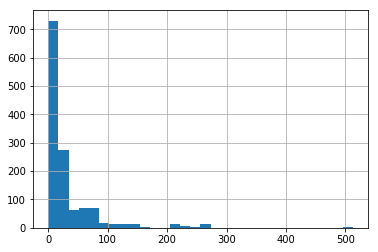There are algorithms for determining an "optimal" number of bins, each of which varies somehow with the number of observations in the data series.

In :
from scipy.stats import kurtosis
doanes = lambda data: int(1 + np.log(len(data)) + np.log(1 + kurtosis(data) * (len(data) / 6.) ** 0.5))

n = len(titanic)
doanes(titanic.fare.dropna())

Out:
14
In :
titanic.fare.hist(bins=doanes(titanic.fare.dropna()))

Out:
<matplotlib.axes._subplots.AxesSubplot at 0x11345dba8>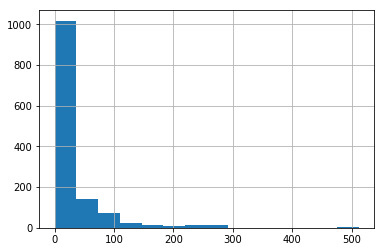A density plot is similar to a histogram in that it describes the distribution of the underlying data, but rather than being a pure empirical representation, it is an estimate of the underlying "true" distribution. As a result, it is smoothed into a continuous line plot. We create them in Pandas using the plot method with kind='kde', where kde stands for kernel density estimate.

In :
titanic.fare.dropna().plot.kde(xlim=(0,600))

Out:
<matplotlib.axes._subplots.AxesSubplot at 0x117655080>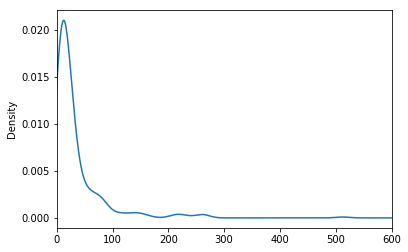Often, histograms and density plots are shown together:

In :
titanic.fare.hist(bins=doanes(titanic.fare.dropna()), normed=True, color='lightseagreen')
titanic.fare.dropna().plot.kde(xlim=(0,600), style='r--')

Out:
<matplotlib.axes._subplots.AxesSubplot at 0x117721048>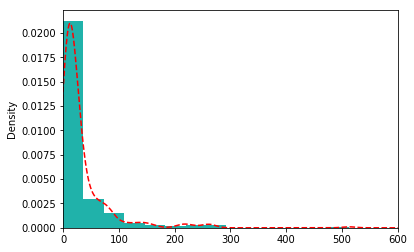Here, we had to normalize the histogram (normed=True), since the kernel density is normalized by definition (it is a probability distribution).

We will explore kernel density estimates more in the next section.

## Boxplots¶

A different way of visualizing the distribution of data is the boxplot, which is a display of common quantiles; these are typically the quartiles and the lower and upper 5 percent values.

In :
titanic.boxplot(column='fare', by='pclass', grid=False)

Out:
<matplotlib.axes._subplots.AxesSubplot at 0x1178e12e8>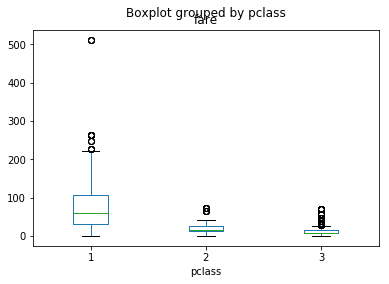You can think of the box plot as viewing the distribution from above. The blue crosses are "outlier" points that occur outside the extreme quantiles.

One way to add additional information to a boxplot is to overlay the actual data; this is generally most suitable with small- or moderate-sized data series.

In :
bp = titanic.boxplot(column='age', by='pclass', grid=False)
for i in [1,2,3]:
y = titanic.age[titanic.pclass==i].dropna()
# Add some random "jitter" to the x-axis
x = np.random.normal(i, 0.04, size=len(y))
plt.plot(x, y.values, 'r.', alpha=0.2)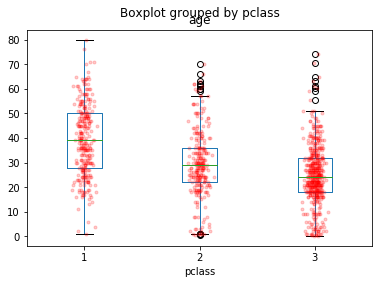When data are dense, a couple of tricks used above help the visualization:

1. reducing the alpha level to make the points partially transparent
2. adding random "jitter" along the x-axis to avoid overstriking

### Exercise¶

Using the Titanic data, create kernel density estimate plots of the age distributions of survivors and victims.

In :
# Write your answer here


## Scatterplots¶

To look at how Pandas does scatterplots, let's look at a small dataset in wine chemistry.

In :
wine = pd.read_table("../data/wine.dat", sep='\s+')

attributes = ['Grape',
'Alcohol',
'Malic acid',
'Ash',
'Alcalinity of ash',
'Magnesium',
'Total phenols',
'Flavanoids',
'Nonflavanoid phenols',
'Proanthocyanins',
'Color intensity',
'Hue',
'OD280/OD315 of diluted wines',
'Proline']

wine.columns = attributes


Scatterplots are useful for data exploration, where we seek to uncover relationships among variables. There are no scatterplot methods for Series or DataFrame objects; we must instead use the matplotlib function scatter.

In :
wine.plot.scatter('Color intensity', 'Hue')

Out:
<matplotlib.axes._subplots.AxesSubplot at 0x117746278>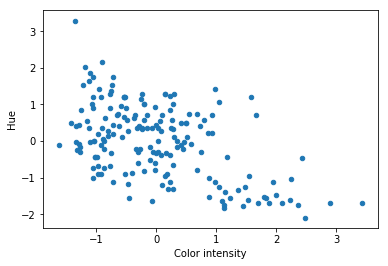We can add additional information to scatterplots by assigning variables to either the size of the symbols or their colors.

In :
wine.plot.scatter('Color intensity', 'Hue', s=wine.Alcohol*100, alpha=0.5)

/Users/fonnescj/anaconda3/envs/dev/lib/python3.6/site-packages/matplotlib/collections.py:865: RuntimeWarning: invalid value encountered in sqrt
scale = np.sqrt(self._sizes) * dpi / 72.0 * self._factor

Out:
<matplotlib.axes._subplots.AxesSubplot at 0x117c29860>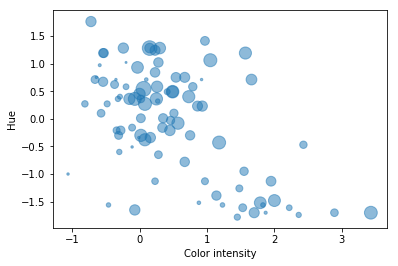In :
wine.plot.scatter('Color intensity', 'Hue', c=wine.Grape)

Out:
<matplotlib.axes._subplots.AxesSubplot at 0x11793c438>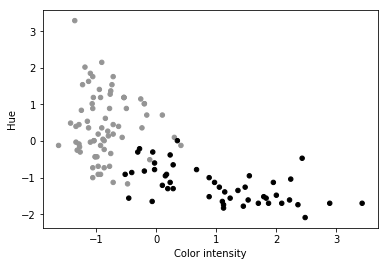In :
wine.plot.scatter('Color intensity', 'Hue', c=wine.Alcohol*100, cmap='hot')

Out:
<matplotlib.axes._subplots.AxesSubplot at 0x117f7ccc0>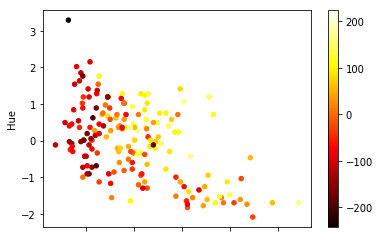To view scatterplots of a large numbers of variables simultaneously, we can use the scatter_matrix function that was recently added to Pandas. It generates a matrix of pair-wise scatterplots, optiorally with histograms or kernel density estimates on the diagonal.

In :
_ = pd.scatter_matrix(wine.loc[:, 'Alcohol':'Flavanoids'], figsize=(14,14), diagonal='kde')

/Users/fonnescj/anaconda3/envs/dev/lib/python3.6/site-packages/ipykernel_launcher.py:1: FutureWarning: pandas.scatter_matrix is deprecated. Use pandas.plotting.scatter_matrix instead
"""Entry point for launching an IPython kernel.